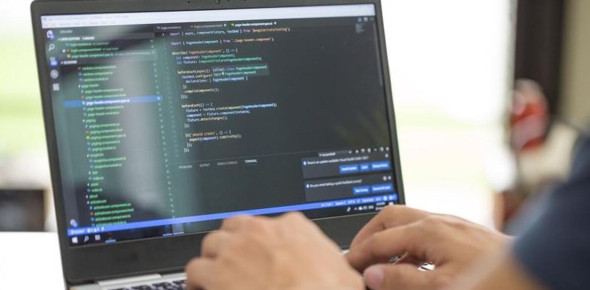# Fundamentals Of Programming For Beginners!

10 Questions | Attempts: 2295
ShareSettingsDo you like making applications or writing code? Are you learning any programming language? If yes, then take this quiz to test your knowledge about the basics of programming. Programming is the art and science of interpreting an algorithm and encoding it into a script. Algorithms are the pivotal part of any programming which find a solution to the problem. Read the questions carefully and answer. Good Luck!

• 1.
Is the following statement true or false? Statement: The default statement in a switch ... case construct has a single break statement.
• A.

True

• B.

False

• 2.
A ______________ construct is used to repeat the execution of a section of a program.
• A.

Decision-making

• B.

Select

• C.

Repeat

• D.

Loop

• 3.
Which of the following are loop constructs? (Choose all that apply)
• A.

Goto

• B.

For loop

• C.

Repeat...while loop

• D.

Repeat...until loop

• E.

While

• 4.
The body of the while loop is continuously executed until the specified condition is _______.
• A.

Completed

• B.

True

• C.

False

• D.

None of the above

• 5.
Is the following statement true or false? Statement: The while loop and repeat...until loop differ only in the way the condition is evaluated
• A.

True

• B.

False

• 6.
What are the three components of the for loop? (Choose all that apply)
• A.

Intialization expression

• B.

Condition expression

• C.

Evaluation Expression

• D.

Increment/Decrement expression

• E.

Result expression

• 7.
Identify whether the following statements are true or false. Statement 1: The initialization in the for statement is done only once. Statement 2: The goto is used when the number of iterations of the loop is known before.
• A.

Both statements are true

• B.

Both statements are false

• C.

Statement 1 is true and Statement 2 is false

• D.

Statement 1 is false and Statement 2 is true

• 8.
There are techniques that provide ways to split a long, continuous program into a series of individual _________ that are related to each other in a specified manner.
• A.

Functions

• B.

Modules

• C.

Procedures

• D.

Subroutines

• E.

Subprograms

• 9.
Which of the following syntax is used to declare a procedure?
• A.

Pro

• B.

Procedure

• C.

Procedure

• D.

Pro

• 10.
What do you call a specific instruction designed to do a task?
• A.

Command

• B.

Process

• C.Back to top